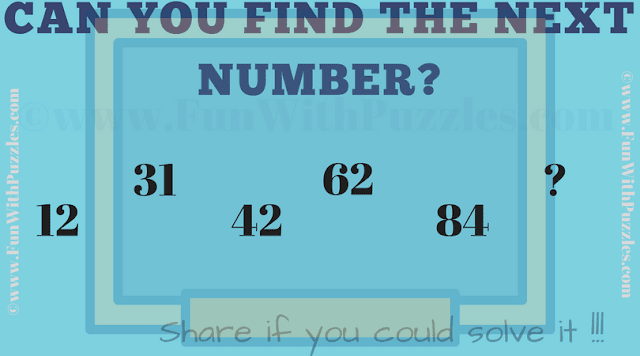This is the tough Math Number Puzzle which will test your brain. In this Math Number Puzzle you are given numbers in a series in the given Puzzle Picture. These numbers relates to each other with some Mathematical or Logical pattern. Your challenge is to decode this hidden relationship between the numbers and then find the value of the next number in the series.Can you find the next number in the series?

Answer of this "Brain Testing Math Number Puzzle", can be viewed by clicking on answer button. Please do give your best try before looking at the answer.

#### 1 comment:

Unknown said...

the answer is 124, AB=A+B A-B